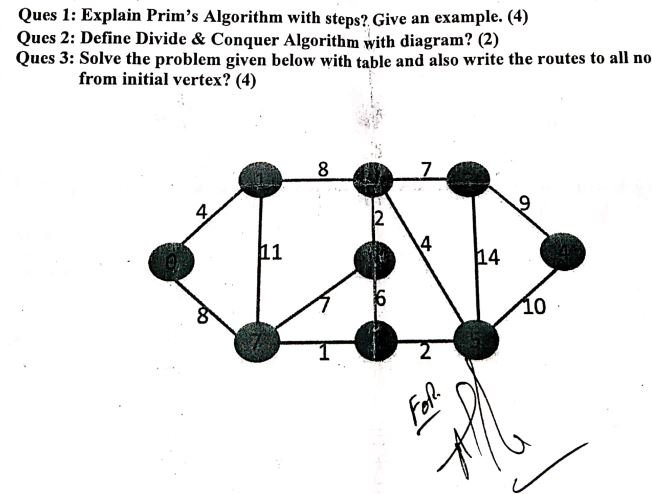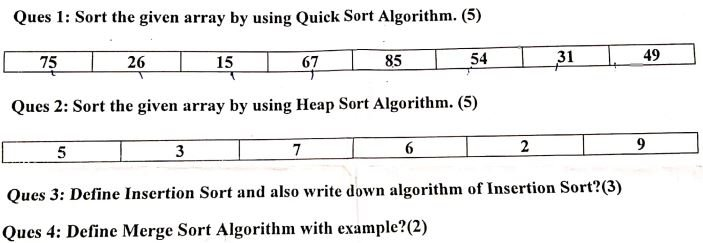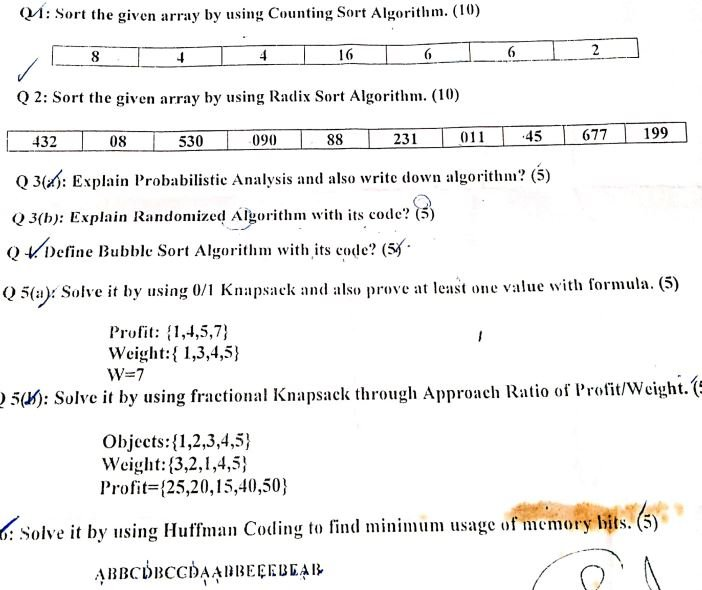# Algorithm past/Previous question paper

#past paper #fyp #final year project #courses #computer science #programming language #project with source code #question paper

-Algorithm project –Computer Science all subject past paper  –Algorithm Problem with source codeAlgorithm Course -Algorithm Project idea -Algorithm MCQ -Algorithm Interview Question  –Computer Science all courses -Technology –University Past Paper -Programming language –Question paperold paper

1. Does input size matter? Suppose a program has run time O(n!) and the run time for n = 10 is 1 second how much it will take for N=16    
1. Write down Algorithm Development Process steps in diagram format. 
2. Running Time of an Algorithm depends on which parameter? 
3. Find time complexity for the following Code 

int j,k;

for (j=0; j<N; j++)

for (k=2; k<8; k++)

sum += k+j;

1. Write a short note on backtracking algorithm 
2. Write short note on the following 
3. In which case Insertion Sort perform Best, Worst and Average time to sort array
4. Write down the name of Linear Time sorting algorithms
5. Why quick sort better than merge sort?
6. In which case quick sort take maximum time to sort array
7. Write down the code for merge sort and discuss it time complexity in detail 
8. Apply the bucket sort algorithm and write down the steps for the following array

0.78  0.17  0.39  0.26  0.72  0.94  0.21  0.12  0.23  0.68#Algorithm project #Computer Science all subject past paper  #Algorithm Problem with source code #Algorithm Course #Algorithm Project idea #Algorithm MCQ #Algorithm Interview Question  #Computer Science all courses #Technology #University Past Paper #Programming language #Question paper #old paper

Scroll to Top